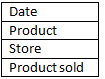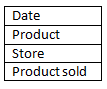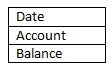# Data Warehousing – Type of facts

There are three types of facts-

Additive- Additive facts are facts that can be added across all the dimensions in the fact table.

Example- Assume for a retail company, we have a fact table with the below attributes:This table is used to record the numbers of product sold for all the stores.

The fact/column product sold can be summed up for each level of its dimensions (date, product, store)

The product sold is called additive fact because it can be summed up though all of the dimension in the fact table.

Non-additive- Non-additive facts are facts that cannot be added for any of the dimensions present in the fact table.

Example- Consider below fact table of a store:Assume that profit for the day 1 is 70% and for day 2 is 30%, so we can’t say that total profit is 100%. In this case                we cannot summed up for any dimensions in the fact table. This is the example of non-additive fact.

Semi-additive- Semi-additive facts are facts that can be added only for some of the dimension tables, but not for all dimensions.

Example- Consider below balance fact table:for a particular day it makes sense to add all the account balance, but it does not make sense to add the balance through time dimension. Here balance is a semi additive fact.

Factless fact- In the real world, it is possible to have a fact table that contains no measures or facts. These tables are called “Factless Fact tables”.

Example – A fact table which has only product key and date key is a factless fact. There are no measures in this table. But still you can get the number products sold over a period of time.

A fact tables that contain aggregated facts are often called summary tables.